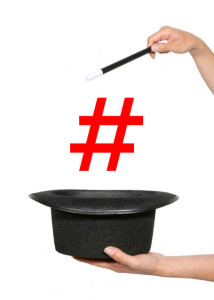# Number magic – Mathematical puzzle

1,230.5K Views

If you multiply me by 2, subtract 1, and read the reverse the result you’ll find me.

Which numbers can I be?37, 397, 3997, 39997, 399…..997 etc.

As
37 * 2 – 1 = 73
397 * 2 – 1 = 793
399….997 * 2 – 1 = 799….993

EXPLANATION-
Method to find the number,
We first try to find the possible number which consist of two digits.

Let the number be x , such that x = AB (where A and B are two digits)

In other words, x = 10 * A + B,
It holds that 2 * x – 1 = 20*A + 2*B – 1

If we reverse this number, we’ll get the original number AB back.
But then it of course also hold that if you reverse the original number (that becomes 10 * B + A), you will get 20*A + 2*B – 1.

Therefore,
20 * A + 2 * B – 1 = 10 * B + A

We can equate this as-
19 * A = 8 * B + 1.

The right hand side of this equation is always odd and hence the left part should be odd as well.
This implies that A should be odd.
The right part is always smaller than 73.

So A cannot be larger than 3.
If we try A = 1,
we do not find a solution.

But A = 3 has a solution with B = 7.
So the number we search for is 37. This is the only number with two digits that satisfies our requirements.Nice answer, but you forgot to include the special case of “1”: 2*1 – 1 = 1.  🙂

on 21st October 2015.Thanks @dougbell, Yup 1 would also be in answer